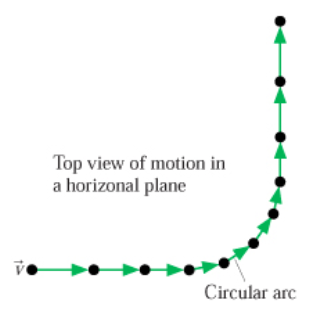# Problem: Complete the motion diagram by adding acceleration vectors. Assume that the dots are numbered from the start point to the end point. Draw the acceleration vectors with their tails at the dots. The orientation of your vectors will be graded. The exact length of your vectors will not be graded.

###### FREE Expert Solution

From the first to the fourth dot, the velocity is decreasing. Thus, the acceleration vector is pointing in the opposite direction relative to the velocity vector.

From the fourth to the eighth dot, the velocity is constant. But the arc is caused by centripetal acceleration.

81% (2 ratings)###### Problem Details

Complete the motion diagram by adding acceleration vectors. Assume that the dots are numbered from the start point to the end point. Draw the acceleration vectors with their tails at the dots. The orientation of your vectors will be graded. The exact length of your vectors will not be graded.Frequently Asked Questions

What scientific concept do you need to know in order to solve this problem?

Our tutors have indicated that to solve this problem you will need to apply the Uniform Circular Motion concept. You can view video lessons to learn Uniform Circular Motion. Or if you need more Uniform Circular Motion practice, you can also practice Uniform Circular Motion practice problems.

What professor is this problem relevant for?

Based on our data, we think this problem is relevant for Professor Johnson's class at APP STATE.